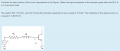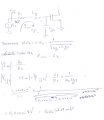# Trying to find the amplitude at the gate of a mosfet

#### asdasd12e12

Joined Nov 24, 2021
48When RLC is in a resonance state that means the total impedance seen by Vs is just Rs. I see no use in this information.

This problem kinda confuses me because there's no current flowing into the gate of this transistor so there would be no voltage drop in Rs so the amplitude at the gate would be the same of signal Vs.

Perhaps if the frequency wasnt the same as the resonance state there would be some flow because of the parasitic capacitance connecting to the source?

Edit, second attempt:Last edited:

#### ronsimpson

Joined Oct 7, 2019
2,515
because there's no current flowing into the gate of this transistor
Why no current flow? There is current flow in the G-S capacitor which is part of the Gate.

#### asdasd12e12

Joined Nov 24, 2021
48
Why no current flow? There is current flow in the G-S capacitor which is part of the Gate.
What path does the corrent that goes through Rs has besides the gate?

#### MisterBill2

Joined Jan 23, 2018
13,689
The current flows in as charge for the gate capacitance. Current is defined as charge flowing. The current flows in to charge the gate capacitance. Because that capacitance must charge before full conduction is achieved is why there is a frequency limit of FET transistor frequency response. And certainly charge must be delivered to charge that capacitance.

We learn that in the AC circuit analysis class, after completing the DC circuit analysis class.

Last edited:

#### ronsimpson

Joined Oct 7, 2019
2,515
What path does the corrent that goes through Rs has besides the gate?
Rs current does flow into the Gate.
At resonant, L&C in series forms a "short" or very close to zero impedance. Vs voltage is across Rs. In the LC there is current but no voltage.

#### crutschow

Joined Mar 14, 2008
31,097
What path does the corrent that goes through Rs has besides the gate?
The gate-source capacitance.

#### MisterBill2

Joined Jan 23, 2018
13,689
See post #4 for the explanation.

#### asdasd12e12

Joined Nov 24, 2021
48
Rs current does flow into the Gate.
At resonant, L&C in series forms a "short" or very close to zero impedance. Vs voltage is across Rs. In the LC there is current but no voltage.
In that case the voltage at the gate is 0V?

#### DickCappels

Joined Aug 21, 2008
9,503
No, the gate has an impedance and current is flowing through it.

The LC looks like a short at resonance, leaving only Rs to limit the current.

What is the resonant frequency?

What is the voltage across Rs?

What is the current through Rs (and thus also through Lg and Vgs)?

What is the impedance of the Gate capacitance at that frequency?

With the known impedance of the gate and the known current, what is the voltage across Vgs?

#### asdasd12e12

Joined Nov 24, 2021
48
No, the gate has an impedance and current is flowing through it.

The LC looks like a short at resonance, leaving only Rs to limit the current.

What is the resonant frequency?

What is the voltage across Rs?

What is the current through Rs (and thus also through Lg and Vgs)?

What is the impedance of the Gate capacitance at that frequency?

With the known impedance of the gate and the known current, what is the voltage across Vgs?
The resonant frequency is sqrt(1/Lg*Cgs) (rads/s) and the current through Rs (and Lg/Vgs) is Us/Rs.

The impedance of the Gate capacitance at that frequency is 1/(j * sqrt(1/L*C) *C) = Xc. With this info the voltage at the gate should be (Us/Rs) * Xc.

One thing that i'm not sure is that if the voltage drop in Rs is Us/Rs * Rs = Us then the voltage at the gate would be negative (still need to consider the voltage drop of Lg), but that doesnt go with the voltage drop of Cgs.

Last edited:

#### asdasd12e12

Joined Nov 24, 2021
48
I added an edit to the main post, i believe that's how we compute the voltage at the gate?

#### Jarvis1.1

Joined Oct 20, 2022
1
View attachment 278880

When RLC is in a resonance state that means the total impedance seen by Vs is just Rs. I see no use in this information.

This problem kinda confuses me because there's no current flowing into the gate of this transistor so there would be no voltage drop in Rs so the amplitude at the gate would be the same of signal Vs.

Perhaps if the frequency wasnt the same as the resonance state there would be some flow because of the parasitic capacitance connecting to the source?

Edit, second attempt:

View attachment 278914
Olha, cuidado que quando fizeste Wo*Cgs, o teu Cgs é 0,75pF

#### DickCappels

Joined Aug 21, 2008
9,503
Note from moderator:

Olha, cuidado que quando fizeste Wo*Cgs, o teu Cgs é 0,75pF
Translation is:

This is an international website, it uses English as its only language. Please post in English only.
Este é um site internacional, usa o inglês como seu único idioma. Por favor, poste apenas em inglês.

#### MisterBill2

Joined Jan 23, 2018
13,689
Electrical resonance in the gate driver circuit is probably something to be avoided because it alters the impedance so much. And probably the exact capacitance is a production variable, with only a range specified in the data sheets.

#### DickCappels

Joined Aug 21, 2008
9,503
Yet, the gate capacitance is intrinsic to this problem. Do you agree with the soltuion?

#### DickCappels

Joined Aug 21, 2008
9,503
The resonant frequency is sqrt(1/Lg*Cgs) (rads/s) and the current through Rs (and Lg/Vgs) is Us/Rs.

The impedance of the Gate capacitance at that frequency is 1/(j * sqrt(1/L*C) *C) = Xc. With this info the voltage at the gate should be (Us/Rs) * Xc.

One thing that i'm not sure is that if the voltage drop in Rs is Us/Rs * Rs = Us then the voltage at the gate would be negative (still need to consider the voltage drop of Lg), but that doesnt go with the voltage drop of Cgs.
The voltage drop across Lg will be equal to the drop across Cgs because the impedances of both are equal. The voltage drop across Rs will be equal to Vs because the impedances of Ls and Cgs will cancel (the series connected impedances will equal a total of zero ohms, leaving only the resistance).

#### WBahn

Joined Mar 31, 2012
27,854
The resonant frequency is sqrt(1/Lg*Cgs) (rads/s) and the current through Rs (and Lg/Vgs) is Us/Rs.

The impedance of the Gate capacitance at that frequency is 1/(j * sqrt(1/L*C) *C) = Xc. With this info the voltage at the gate should be (Us/Rs) * Xc.
You've got it. Except you are getting sloppy with your math equations. The expression sqrt(1/Lg*Cgs) means sqrt((1/Lg)*Cgs) whereas you meant sqrt(1/(Lg*Cgs)). Those parens can't be left out. You really want to get in the habit of being precise about order of operations. Yes, most of us can read your mind and quickly figure out what you meant, but sooner or later you WILL get bit when you type an expression into a program or spreadsheet and unconsciously be expecting it to read your mind.

One thing that i'm not sure is that if the voltage drop in Rs is Us/Rs * Rs = Us then the voltage at the gate would be negative (still need to consider the voltage drop of Lg), but that doesnt go with the voltage drop of Cgs.
At resonance, there is still a voltage across the inductor and a voltage across the capacitor, but they are 180° out of phase and are equal in magnitude, so when you look across the combination of the two, you see no voltage, but if you look at each one individually, you can see significant voltage. In fact, you can see quite a bit more voltage across each than the supply voltage (consider the case if Rs << Xc).

#### WBahn

Joined Mar 31, 2012
27,854
Electrical resonance in the gate driver circuit is probably something to be avoided because it alters the impedance so much. And probably the exact capacitance is a production variable, with only a range specified in the data sheets.
Whether it is to be avoided or not is really not the issue for this problem, which seems to be exploring the question of what can happen with the parasitic trace capacitance IS in resonance with the gate capacitance.